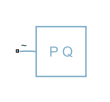Since R2020b

•Libraries:
Simscape / Electrical / Passive

## Description

The Constant Power Load (Three-Phase) block implements a constant power load for a three-phase supply.

The block outputs a nominal rated power as long as the voltage from the three-phase supply is equal to or greater than the value specified for the Minimum supply voltage (phase-to-phase RMS) parameter.

When the voltage from the three-phase supply drops below the value of Minimum supply voltage (phase-to-phase RMS), the load behaviour changes and the block models a load with constant impedance.

### Equations

When the voltage from the three-phase supply is greater than the value specified for the Minimum supply voltage (phase-to-phase RMS) parameter, the block acts in constant PQ mode.

The active and reactive power are defined by:

`$\begin{array}{l}P=\frac{3}{2}{V}_{pk}{I}_{pk}cos\left({\phi }_{V}-{\phi }_{I}\right)\\ Q=\frac{3}{2}{V}_{pk}{I}_{pk}\mathrm{sin}\left({\phi }_{V}-{\phi }_{I}\right)\end{array}$`

where:

• Vpk is the voltage peak magnitude.

• ${\phi }_{I}={\phi }_{V}-atan\left(\frac{Q}{P}\right)$ is the phase of the current.

• ${I}_{pk}=\frac{2}{3}\frac{P}{{V}_{pk}cos\left({\phi }_{V}-{\phi }_{I}\right)}$ is the current peak magnitude.

During the first period, the peak current is equal to 0 A.

When the voltage from the three-phase supply is less than or equal to the value specified for the Minimum supply voltage (phase-to-phase RMS) parameter, the block acts in constant Z mode.

The active and reactive power are defined by:

`$\begin{array}{l}P=\frac{3}{2}{V}_{pk}^{2}\frac{R}{{R}^{2}+{X}^{2}}\\ Q=\frac{3}{2}{V}_{pk}^{2}\frac{X}{{R}^{2}+{x}^{2}}\end{array}$`

where:

• $R={V}_{line\text{\hspace{0.17em}}RM{S}_{min}}\frac{P}{{P}^{2}+{Q}^{2}}$ is the constant resistance.

• $X={V}_{line\text{\hspace{0.17em}}RM{S}_{min}}\frac{Q}{{P}^{2}+{Q}^{2}}$ is the constant reactance.

• Vline RMSmin is the Minimum supply voltage (phase-to-phase RMS).

If the block is in a network that is compatible with frequency-time simulation mode, you can perform a load-flow analysis on the network. A load-flow analysis provides steady-state values that you can use to initialize a machine.

For more information, see Perform a Load-Flow Analysis Using Simscape Electrical and Frequency and Time Simulation Mode.

### Faults

To model a fault in the Constant Power Load (Three-Phase) block, in the Faults section, click the Add fault hyperlink in the parameter that corresponds to the specific fault that you want to model. When the Create Fault window opens, you use it to specify the fault properties. For more information about fault modeling, see Fault Behavior Modeling and Fault Triggering.

The Constant Power Load (Three-Phase) block allows you to model an electrical fault as an open circuit. The block can trigger fault events at a specific time.

## Ports

### Conserving

expand all

Composite three-phase port associated with the three-phase voltage.

#### Dependencies

To enable this port, set Electrical connection to ```Composite three-phase ports```.

Expanded three-phase port associated with the phase A voltage.

#### Dependencies

To enable this port, set Electrical connection to ```Expanded three-phase ports```.

Expanded three-phase port associated with the phase B voltage.

#### Dependencies

To enable this port, set Electrical connection to ```Expanded three-phase ports```.

Expanded three-phase port associated with the phase C voltage.

#### Dependencies

To enable this port, set Electrical connection to ```Expanded three-phase ports```.

## Parameters

expand all

### Main

Whether to model composite or expanded three-phase ports.

Composite three-phase ports represent three individual electrical conserving ports with a single block port. You can use composite three-phase ports to build models that correspond to single-line diagrams of three-phase electrical systems.

Expanded three-phase ports represent the individual phases of a three-phase system using three separate electrical conserving ports.

Since R2023a

Rated electrical frequency.

Active power consumed by the block.

Reactive power consumed by the block.

Minimum voltage, in phase-to-phase RMS, that the supply needs for the block to act as a constant power load.

Since R2023a

Time constant associated with the transients of the terminal voltage measurement.

Since R2023a

Terminal voltage, in phase-to-phase RMS, at time zero.

Since R2023a

Terminal voltage angle of phase A at time zero.

Since R2023a

Parasitic conductance to electrical reference.

### Faults

Option to add an open-circuit fault in the Constant Power Load (Three-Phase) block.

Conductance during the open-circuit state.

#### Dependencies

To enable this parameter, add a fault to the Constant Power Load (Three-Phase) block by clicking the Add fault hyperlink in the Open circuit fault parameter.

After you create the fault, you can change the properties in the Fault Inspector window. When you open a block that has a fault, the Open Fault Inspector hyperlink appears instead of the Add fault hyperlink. For an example that shows how to include faults, see Analyze a DC Armature Winding Fault.

Simulation time at which the block enters the faulted state.

#### Dependencies

To enable this parameter, in the Fault Inspector window, set Trigger Type to `Timed`.

This parameter appears in the Trigger section of the Fault Inspector window. For more information, see Set Fault Triggers.

## Version History

Introduced in R2020b

expand all